Olympiad Test: Embedded Figures - 2

# Olympiad Test: Embedded Figures - 2

Test Description

## 10 Questions MCQ Test National Cyber Olympiad Class 6 | Olympiad Test: Embedded Figures - 2

Olympiad Test: Embedded Figures - 2 for Class 6 2023 is part of National Cyber Olympiad Class 6 preparation. The Olympiad Test: Embedded Figures - 2 questions and answers have been prepared according to the Class 6 exam syllabus.The Olympiad Test: Embedded Figures - 2 MCQs are made for Class 6 2023 Exam. Find important definitions, questions, notes, meanings, examples, exercises, MCQs and online tests for Olympiad Test: Embedded Figures - 2 below.
 1 Crore+ students have signed up on EduRev. Have you?
Olympiad Test: Embedded Figures - 2 - Question 1

### Directions: In each of the following questions, you are given a figure (X) followed by four alternative figures (A), (B), (C) and (D) such that figure (X) is embedded in one of them. Find out the alternative figure which contains fig. (X) as its part. Q. Find out the alternative figure which contains figure (X) as its part.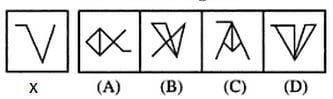Olympiad Test: Embedded Figures - 2 - Question 2

### Directions: In each of the following questions, you are given a figure (X) followed by four alternative figures (A), (B), (C) and (D) such that figure (X) is embedded in one of them. Find out the alternative figure which contains fig. (X) as its part. Q. Find out the alternative figure which contains figure (X) as its part.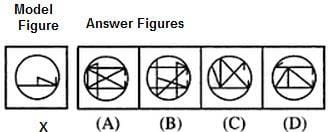Olympiad Test: Embedded Figures - 2 - Question 3

### Directions: In each of the following questions, you are given a figure (X) followed by four alternative figures (A), (B), (C) and (D) such that figure (X) is embedded in one of them. Find out the alternative figure which contains fig. (X) as its part. Q. Find out the alternative figure which contains figure (X) as its part.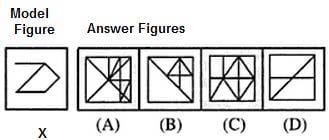Olympiad Test: Embedded Figures - 2 - Question 4

Directions: In each of the following questions, you are given a figure (X) followed by four alternative figures (A), (B), (C) and (D) such that figure (X) is embedded in one of them. Find out the alternative figure which contains fig. (X) as its part.

Q. Find out the alternative figure which contains figure (X) as its part.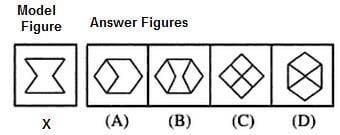Olympiad Test: Embedded Figures - 2 - Question 5

Directions: In each of the following questions, you are given a figure (X) followed by four alternative figures (A), (B), (C) and (D) such that figure (X) is embedded in one of them. Find out the alternative figure which contains fig. (X) as its part.

Q. Find out the alternative figure which contains figure (X) as its part.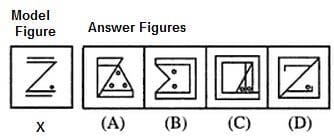Olympiad Test: Embedded Figures - 2 - Question 6

Directions: In each of the following questions, you are given a figure (X) followed by four alternative figures (A), (B), (C) and (D) such that figure (X) is embedded in one of them. Find out the alternative figure which contains fig. (X) as its part.

Q. Find out the alternative figure which contains figure (X) as its part.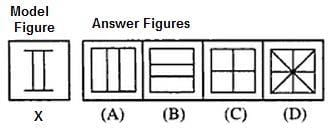Olympiad Test: Embedded Figures - 2 - Question 7

Directions: In each of the following questions, you are given a figure (X) followed by four alternative figures (A), (B), (C) and (D) such that figure (X) is embedded in one of them. Find out the alternative figure which contains fig. (X) as its part.

Q. Find out the alternative figure which contains figure (X) as its part.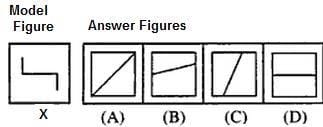Olympiad Test: Embedded Figures - 2 - Question 8

Directions: In each of the following questions, you are given a figure (X) followed by four alternative figures (A), (B), (C) and (D) such that figure (X) is embedded in one of them. Find out the alternative figure which contains fig. (X) as its part.

Q. Find out the alternative figure which contains figure (X) as its part.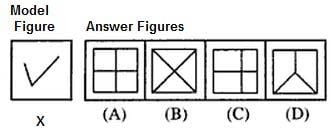Olympiad Test: Embedded Figures - 2 - Question 9

Directions: In each of the following questions, you are given a figure (X) followed by four alternative figures (A), (B), (C) and (D) such that figure (X) is embedded in one of them. Find out the alternative figure which contains fig. (X) as its part.

Q. Find out the alternative figure which contains figure (X) as its part.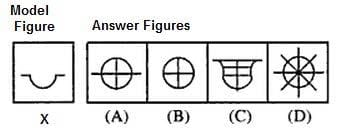Olympiad Test: Embedded Figures - 2 - Question 10

Directions: In each of the following questions, you are given a figure (X) followed by four alternative figures (A), (B), (C) and (D) such that figure (X) is embedded in one of them. Find out the alternative figure which contains fig. (X) as its part.

Q. Find out the alternative figure which contains figure (X) as its part.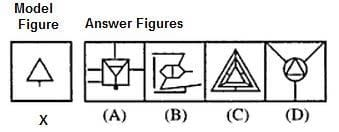## National Cyber Olympiad Class 6

34 tests
 Use Code STAYHOME200 and get INR 200 additional OFF Use Coupon Code